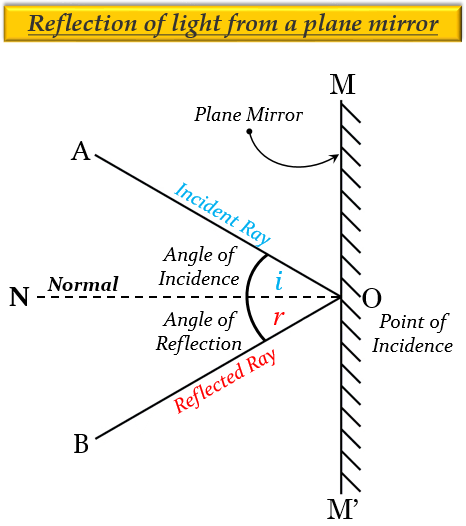# Angle of incidence is equal to the angle of reflection.$(a)$. Always$(b)$. Sometimes$(c)$. Under special conditions $(d)$. Never

$(a)$. Always

Explanation

There are two laws of reflection which are as follows:

1. The angle of incidence (∠i) is always equal to the angle of reflection (∠r), which is given as, ∠i = ∠rFor example, the angle of reflection NOB is exactly equal to the angle of incidence AON in the figure given below.

2. The incident ray, the reflected ray and the normal (at the point of incidence), all lie in the same plane. For example, in the figure given below, the incident ray AO, the reflected ray OB and the normal ON, all lie in the same plane.